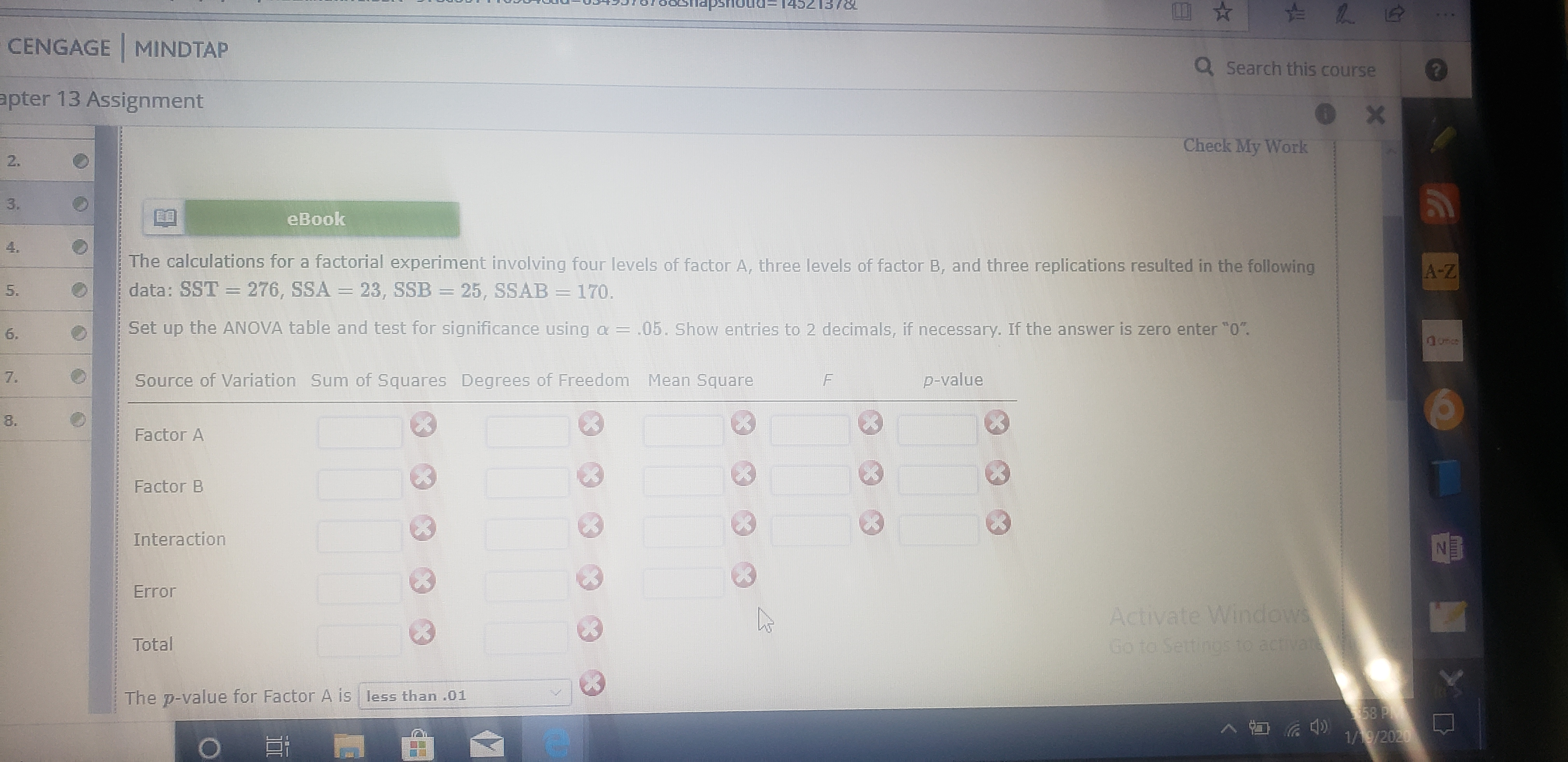# nousdeCENGAGE MINDTAPQ Search this courseapter 13 AssignmentCheck My Work2.3.eBook4.The calculations for a factorial experiment involving four levels of factor A, three levels of factor B, and three replications resulted in the followingA-Zdata: SST = 276, SSA = 23, SSB = 25, SSAB = 170.5.Set up the ANOVA table and test for significance using a= .05. Show entries to 2 decimals, if necessary. If the answer is zero enter "0".1.Source of Variation Sum of Squares Degrees of FreedomMean Squarep-value8.Factor AFactor BInteractionNEErrorActivate WindowsTotalGo to Settings to activateThe p-value for Factor A is less than .0158 P1/9/2020LL6.7,

Question
109 views

The caluations for a factorial experiment involving four levels of factor A, three levels of factor B, and three replications resulting in the following data: SST = 276, SSA=23, SSB = 25, SSAB =170.

Set up the ANOVA table and test for significance using a=0.5. Show entries to 2 decimals, if necessary.  If the answer is zeeo enter 0.

Factor A?

Factor B?

Interaction?

Error?

Total?

Thank youhelp_outlineImage Transcriptionclosenousde CENGAGE MINDTAP Q Search this course apter 13 Assignment Check My Work 2. 3. eBook 4. The calculations for a factorial experiment involving four levels of factor A, three levels of factor B, and three replications resulted in the following A-Z data: SST = 276, SSA = 23, SSB = 25, SSAB = 170. 5. Set up the ANOVA table and test for significance using a= .05. Show entries to 2 decimals, if necessary. If the answer is zero enter "0". 1. Source of Variation Sum of Squares Degrees of Freedom Mean Square p-value 8. Factor A Factor B Interaction NE Error Activate Windows Total Go to Settings to activate The p-value for Factor A is less than .01 58 P 1/9/2020 LL 6. 7, fullscreen
check_circle

Step 1

According to the provided information,

Step 2

ANOVA Table can be calculated as:

Step 3

Here,

...

### Want to see the full answer?

See Solution

#### Want to see this answer and more?

Solutions are written by subject experts who are available 24/7. Questions are typically answered within 1 hour.*

See Solution
*Response times may vary by subject and question.
Tagged in
MathStatistics

### Other